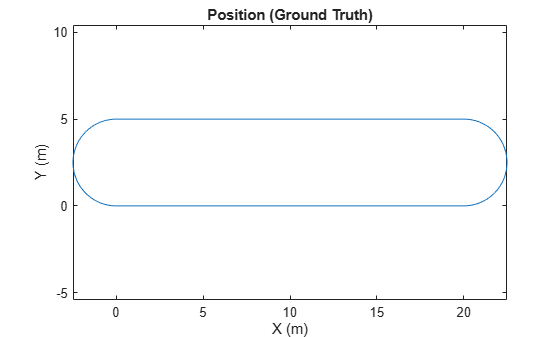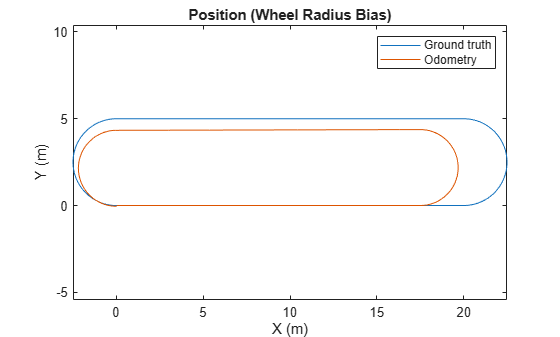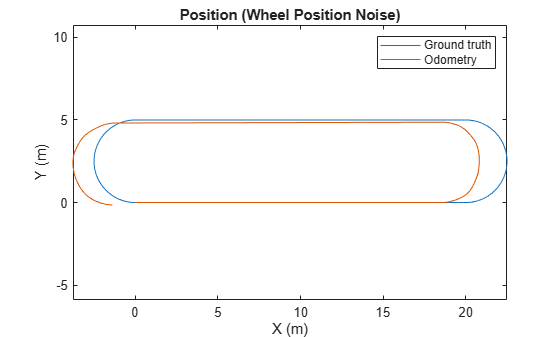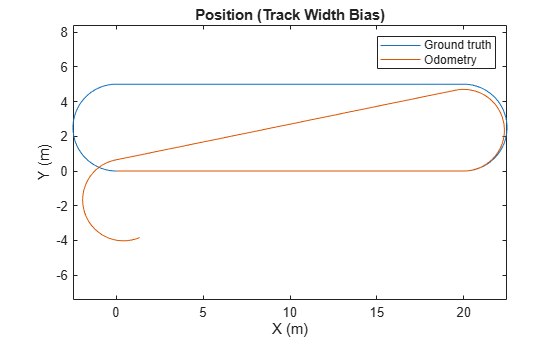# Wheel Encoder Error Sources

Explore the various error sources of wheel encoders and how they affect the wheel odometry estimate. After defining a ground truth trajectory, change parameters for wheel radius bias, wheel position noise, wheel slippage, and track width for the various wheel encoder objects. Notice the affects of changing these parameters on the output trajectory from the wheel encoder sensor models.

### Ground Truth Trajectory

Create a ground truth trajectory to be used when examining the error parameters. Plot the trajectory.

```Fs = 100; wps = [0 0 0; 20 0 0; 20 5 0; 0 5 0; 0 0 0]; toa = cumsum([0 10 1.25*pi 10 1.25*pi]).'; vels = [2 0 0; 2 0 0; -2 0 0; -2 0 0; 2 0 0]; traj = waypointTrajectory(wps,... 'SampleRate',Fs,'ReferenceFrame','ENU', ... 'TimeOfArrival',toa,'Velocities',vels); % Fetch pose values. [pos,orient,vel,acc,angvel] = lookupPose(traj,toa(1):1/Fs:toa(end)); angvelBody = rotateframe(orient,angvel); % Plot ground truth position. figure plot(pos(:,1),pos(:,2)) title('Position (Ground Truth)') xlabel('X (m)') ylabel('Y (m)') axis equal```### Bias in the Wheel Radius

Create a wheel encoder sensor for a unicycle model as a `wheelEncoderUnicycle` object. Specify a non-zero `WheelRadiusBias` and examine how it affects the odometry estimate. Specifying a positive bias causes the odometry algorithm to underestimate the circumference of the wheel. This results in the odometry estimating a smaller distance traveled.

```encoder = wheelEncoderUnicycle; encoder.WheelRadiusBias = 0.05; odom = wheelEncoderOdometryUnicycle(encoder); ticks = encoder(vel, angvel, orient); estPose = odom(ticks, angvelBody(:,3)); % Plot ground truth and estimated positions. figure plot(pos(:,1),pos(:,2),estPose(:,1),estPose(:,2)) title('Position (Wheel Radius Bias)') xlabel('X (m)') ylabel('Y (m)') legend('Ground truth','Odometry') axis equal```### Noise in the Wheel Position Measurement

Specify a non-zero `WheelPositionAccuracy` and examine how it affects the odometry estimate.This noise adds random deviations to the measured ticks from the wheel encoder.

```encoder = wheelEncoderUnicycle; encoder.WheelPositionAccuracy = 0.1; % Use a local random stream to reproduce results. encoder.RandomStream = 'mt19937ar with seed'; odom = wheelEncoderOdometryUnicycle(encoder); ticks = encoder(vel,angvel,orient); estPose = odom(ticks,angvelBody(:,3)); % Plot ground truth and estimated positions. figure plot(pos(:,1),pos(:,2),estPose(:,1),estPose(:,2)) title('Position (Wheel Position Noise)') xlabel('X (m)') ylabel('Y (m)') legend('Ground truth', 'Odometry') axis equal```### Wheel Slippage and Skidding

Specify a non-zero `SlipRatio` and examine how it affects the odometry estimate. Specifying a value greater than zero simulates wheel slipping. This slippage results in the odometry estimating a larger distance traveled. A negative value for slip ratio inidates skidding.

```encoder = wheelEncoderUnicycle; encoder.SlipRatio = 0.25; odom = wheelEncoderOdometryUnicycle(encoder); ticks = encoder(vel, angvel, orient); estPose = odom(ticks, angvelBody(:,3)); % Plot ground truth and estimated positions. figure plot(pos(:,1),pos(:,2),estPose(:,1),estPose(:,2)) title('Position (Wheel Slippage)') xlabel('X (m)') ylabel('Y (m)') legend('Ground truth', 'Odometry') axis equal```### Bias in the Track Width

Specify a non-zero `TrackWidthBias` and examine how it affects the odometry estimate.Specifying a positive bias will cause the odometry algorithm to overestimate the turning angle of the vehicle. This overestimation of turning results in drift acumulating in the odometry estimate at turns. For this scenario, a vehicle with an axle is needed, so use the `wheelEncoderDifferentialDrive` object.

```encoder = wheelEncoderDifferentialDrive; encoder.TrackWidthBias = 0.1; odom = wheelEncoderOdometryDifferentialDrive(encoder); ticks = encoder(vel,angvel,orient); estPose = odom(ticks); % Plot ground truth and estimated positions. figure plot(pos(:,1),pos(:,2), estPose(:,1), estPose(:,2)) title('Position (Track Width Bias)') xlabel('X (m)') ylabel('Y (m)') legend('Ground truth', 'Odometry') axis equal```### Differing Biases in the Wheels

Specify different non-zero values for the `WheelRadiusBias` and examine how it affects the odometry estimate.Specifying different biases causes the odometry estimate to drift throughout the entire trajectory. For this scenario, two wheels are needed, so use the `wheelEncoderDifferentialDrive` object.

```encoder = wheelEncoderDifferentialDrive; encoder.WheelRadiusBias = [-0.01, 0.001]; odom = wheelEncoderOdometryDifferentialDrive(encoder); ticks = encoder(vel, angvel, orient); estPose = odom(ticks); % Plot ground truth and estimated positions. figure plot(pos(:,1),pos(:,2), estPose(:,1), estPose(:,2)) title('Position (Different Wheel Biases)') xlabel('X (m)') ylabel('Y (m)') legend('Ground truth', 'Odometry') axis equal```